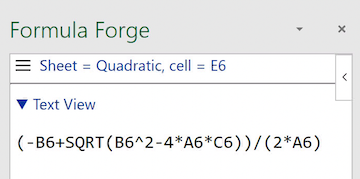# The Text View

The text view displays the text of a formula, just it appears in Excel's formula bar, but without the leading `=` sign. For example, here is how the text view displays the familiar quadratic formula using Excel's notations for powers and square roots.Text view of the quadratic formula